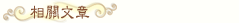# 如何讀懂「數學英文」來解答出數學應用題

人氣: 448
【字號】
標籤:

【大紀元2016年05月19日訊】學生們在解答數學應用題時常常在想：「怎樣才能最好的理解這道題的題意，並把它分解成幾個簡單的問題？」「如何把這個包含數字的句子轉換成我能理解的英文？」本文就來討論如何破除數學應用題的神秘感。

Solving a mixture problem takes precision, but you can break it down with mathematical English as well. You have to remember these steps. 1) What is being mixed? 2) Organize the information. 3) Set up gallons of the ingredient for each container. 4) Form a mixture equation. 5) Solve and Check.

Q: How many gallons of a 60% solution must be added to 30 gallons of a 10% solution in order to produce a 20% solution?

We know so far that a 60% solution is being mixed with a 10% solution. We do NOT know how much of the 60% solution we have, so that is “x”. We do have 30 gallons of the 10% solution.

The next step is to ORGANIZE the information. One jar contains the 60% solution (x gallons). Another jar contains the 10% solution (30 gallons). There is a 20% solution jar that combines the two as well (x + 30). The last jar is the place where we want to have the 20% solution created. In the first jar, we have a 60% solution that houses 60% of the gallons of one liquid. In the other jar, we have 40% of the container having other ingredients.

Now, it is time to calculate the gallons of the ingredient in each of the containers by multiplying the total gallons of EACH container by the percentage listed for the container.   Next, you have 60% * x, 10% * 30, and 20% * (x+30). The verbal equation is (Ingredient in Jar 1) + (Ingredient in Jar 2) = (Ingredient in Jar 3). The equation using decimals is: .60x + 3 = .20x + 6. You would then subtract .20x from both sides and subtract 3 from both sides to get .40x = 3. Next, you divide both sides by .40 and x = 7.5.

Problem solved and you should check it by plugging the value for x back into the equation.  This is how easy math can be using mathematical English.   Let’s try this again in a different way.

“What is 75% of 300?”

How are we going to break this problem down? When a problem says “What”, that means “x”. The word “is” ALWAYS means “equals”. “Percentage” always means “out of 100 parts”, so the 75% converts to 75/100. “Of” means multiplication and the number 300 remains just that – a number. So, here is the updated version of this: x = (75/100) * 300. Now, 100 goes into 300 three times. So, you have 3 times 75 which equals 225. Problem solved.  This is how easy math can be using mathematical English. Let’s try this again in a different way.

35 is what percent of 80?

We now solve this problem: 80 goes into 100 1.25 times. So, we have 35 = x/1.25. We then cross multiply 35 * 1.25 and get 43.75 which is our answer. X = 43.75%. As you see, this process is again very simple. Visual learners will need to break problems down by drawing pictures or sketching out the process of the problem. Auditory learners just need to hear the correct conversion of the problem. Kinesthetic learners will need to get up and act out the problem or write on the boards themselves. This simple mathematical English process can be used on more advanced problems to provide clarity to the problem solver.• 美國大學理事會（College Board）去年4月公布「學術水平測驗考試」（SAT，俗稱美國高考）考試改革，全美報考週六（3月5日）SAT考試的高中生，將是新SAT的首批考生。在此特別提醒考生，新SAT的改變，以及非入學目的的考生，下次再考。
• 在美國的中國留學生人數已突破30萬，遠超過第2名的印度。隨著中國留學生的增多，美國各大學教授發現，評量成績優異的中國學生，來美國後在課堂上的表現與美國學生大不同，普遍欠缺批判性思維，無法完成分析性寫作，也不知如何與同學及教授交談。哥倫比亞大學教授林曉東（Lin Xiaodong，音譯）認為，這是因為中國大陸的教育偏重灌輸知識，而美國教育則強調如何學習。
• 俗稱「美國高考」、大陸學生赴美留學必考的SAT考試，每年吸引數以十萬計中國學生湧入香港應考，還催生一個產值過億的販賣試題「灰色產業鏈」。今年1月，主辦單位已因試題外洩，取消在大陸和澳門的45場SAT考試。而最近改革的新SAT將於5月7日在香港進行亞洲首考，不過，外媒報導新題目已經外洩，本報調查多個來港報考團，發現不少大陸培訓中心都以「真試題」招徠考生。香港SAT考試能否經得起「作弊」考驗，備受關注。
• 日前大陸胡潤百富發布的《2016留學趨勢特別報告》告顯示，近幾年中國學生海外留學的年均增長率為19.06%。造成這個留學趨勢的原因，大多數是為了逃避大陸僵化的高考制度，選擇海外自由開放的教育體制，就讀符合自己興趣的學科。
• 根據對法國全國綜合大學本科第一年申請志願的多少，得出法國最受歡迎的20所綜合大學排名，其中巴黎索邦大學（Paris 1 Panthéon Sorbonne）位居榜首。《費加羅報》同時還分析了20所綜合大學學士第一年招生名額占申報人數的比率，從而可看出申請這些學校的錄取機率。
• 2020年以前，聯邦教育部和ACAOM（針灸和東方醫學認證委員會）對中醫學科入學時的英語成績要求是托福45分以上，而到了2020年，中醫學科英語入學成績要求托福80分以上！要求顯著提高。有志於在美國做一名中醫師、而英語又不太好的學生得抓緊時間報名了！